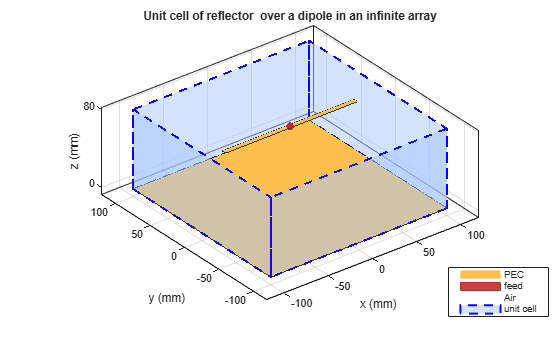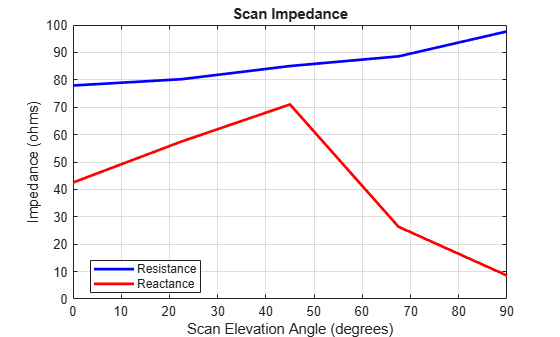infiniteArray

Create 2-D custom mesh antenna on X-Y plane

Description

The infiniteArray object is an infinite antenna array in the X-Y plane. Infinite array models a single antenna element called the unit cell. Ground plane of the antennas specifies the boundaries of the unit cell. Antennas without a ground plane require a reflector. By default, the infinite array has reflector-backed dipoles as antenna elements. The default dimensions are chosen for an operating frequency of 1 GHz.Creation

Description

infa = infiniteArray creates an infinite antenna array in the X-Y plane.

example

infa = infiniteArray(Name,Value) creates an infinite antenna array with additional properties specified by one, or more name-value pair arguments. Name is the property name and Value is the corresponding value. You can specify several name-value pair arguments in any order as Name1, Value1, ..., NameN, ValueN. Properties not specified retain default values.

Properties

expand all

Type of individual antenna elements in unit cell, specified as an object. Antenna without a groundplane is backed using a reflector. The ground plane size specifies the unit cell boundaries.

Example: 'Element',reflector

Scan direction in azimuth plane, specified as a scalar in degrees.

Example: 'ScanAzimuth',25

Data Types: double

Scan direction in elevation plane, specified as a scalar in degrees.

Example: 'ScanElevation',80

Data Types: double

Remove the ground plane of the reflector with air substrate, specified as a numeric or logical 1 (true) or 0 (false). When you specify 1, the object removes the ground plane. When you specify 0, the object does not remove the ground plane

Example: 'RemoveGround',1

Data Types: logical

Object Functions

 numSummationTerms Change number of summation terms for calculating periodic Green's function show Display antenna or array structure; display shape as filled patch info Display information about antenna or array axialRatio Axial ratio of antenna beamwidth Beamwidth of antenna charge Charge distribution on metal or dielectric antenna or array surface correlation Correlation coefficient between two antennas in array current Current distribution on metal or dielectric antenna or array surface design Design prototype antenna or arrays for resonance around specified frequency EHfields Electric and magnetic fields of antennas; Embedded electric and magnetic fields of antenna element in arrays impedance Input impedance of antenna; scan impedance of array layout Display array or PCB stack layout mesh Mesh properties of metal or dielectric antenna or array structure pattern Radiation pattern and phase of antenna or array; Embedded pattern of antenna element in array patternAzimuth Azimuth pattern of antenna or array patternElevation Elevation pattern of antenna or array rcs Calculate and plot radar cross section (RCS) of platform, antenna, or array returnLoss Return loss of antenna; scan return loss of array sparameters Calculate S-parameter for antenna and antenna array objects

Examples

collapse all

Create an infinite array with reflector-backed dipoles as unit cells. Scan the array at boresight. Visualize the unit cell.

infa = infiniteArray('Element',reflector,'ScanAzimuth',0, ...
'ScanElevation',90);
show(infa)Calculate the scan impedance of an infinite array at 1GHz. To calculate the impedance, scan the infinite array from boresight to horizon in the elevation plane.

infa = infiniteArray;
theta0deg = linspace(0,90,5);
zscan = nan(1,numel(theta0deg));
for j = 1:numel(theta0deg)
infa.ScanElevation = theta0deg(j);
zscan(1,j) = impedance(infa,1e9);
end
plot(zscan)Calculate the scan impedance at bore sight of a metal infinite array without ground plane at a frequency of 1 GHz.

h = infiniteArray('Element', reflector, 'ScanAzimuth', 0, ...
'ScanElevation', 90, 'RemoveGround', 1);
zin = impedance(h,1e9)
zin = 48.2919 + 13.1800i
impedance(h,1e9)Plot the scan impedance at the boresight of a metal-dielectric infinite array at a frequency of 1 GHz.

ant = patchMicrostrip('Substrate',dielectric('Teflon'));
h = infiniteArray('Element',ant,'ScanAzimuth',0,'ScanElevation',90);
impedance(h,1e9)Balanis, C.A. Antenna Theory: Analysis and Design. 3rd Ed. New York: Wiley, 2005.